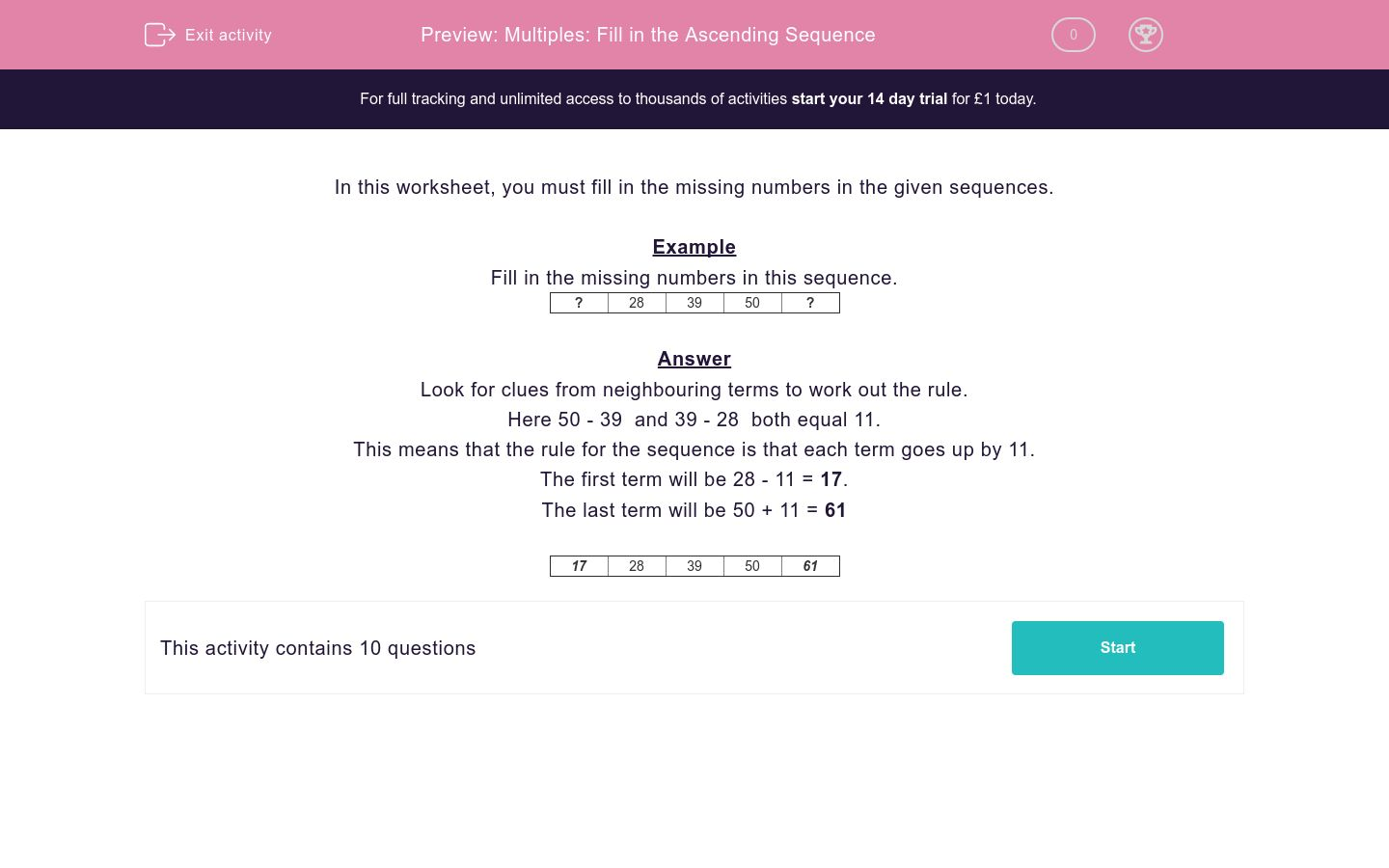# Multiples: Fill in the Ascending Sequence

In this worksheet, students fill in the missing numbers of a sequence by first working out the rule.Key stage:  KS 2

Curriculum topic:   Maths and Numerical Reasoning

Curriculum subtopic:   Sequences

Difficulty level:### QUESTION 1 of 10

In this worksheet, you must fill in the missing numbers in the given sequences.

Example

Fill in the missing numbers in this sequence.

 ? 28 39 50 ?

Look for clues from neighbouring terms to work out the rule.

Here 50 - 39  and 39 - 28  both equal 11.

This means that the rule for the sequence is that each term goes up by 11.

The first term will be 28 - 11 = 17.

The last term will be 50 + 11 = 61

 17 28 39 50 61

Fill in the missing numbers in this sequence.

 50 65 A B 110
 Missing Numbers A B

Fill in the missing numbers in this sequence.

 23 36 49 A B
 Missing Numbers A B

Fill in the missing numbers in this sequence.

 26 A B 56 66
 Missing Numbers A B

Fill in the missing numbers in this sequence.

 37 46 55 A B
 Missing Numbers A B

Fill in the missing numbers in this sequence.

 28 37 A 55 B
 Missing Numbers A B

Fill in the missing numbers in this sequence.

 A B 71 83 95
 Missing Numbers A B

Fill in the missing numbers in this sequence.

 A B 49 58 67
 Missing Numbers A B

Fill in the missing numbers in this sequence.

 A 37 B 59 70
 Missing Numbers A B

Fill in the missing numbers in this sequence.

 A 46 B 70 82
 Missing Numbers A B

Fill in the missing numbers in this sequence.

 45 A B C 81

## Column B

A
72
B
54
C
63
• Question 1

Fill in the missing numbers in this sequence.

 50 65 A B 110
 Missing Numbers A B
EDDIE SAYS
The sequence goes up by 15 each time.
• Question 2

Fill in the missing numbers in this sequence.

 23 36 49 A B
 Missing Numbers A B
EDDIE SAYS
The sequence goes up by 13 each time.
• Question 3

Fill in the missing numbers in this sequence.

 26 A B 56 66
 Missing Numbers A B
EDDIE SAYS
The sequence goes up by 10 each time.
• Question 4

Fill in the missing numbers in this sequence.

 37 46 55 A B
 Missing Numbers A B
EDDIE SAYS
The sequence goes up by 9 each time.
• Question 5

Fill in the missing numbers in this sequence.

 28 37 A 55 B
 Missing Numbers A B
EDDIE SAYS
This sequence also goes up by 9 each time.
• Question 6

Fill in the missing numbers in this sequence.

 A B 71 83 95
 Missing Numbers A B
EDDIE SAYS
This sequence goes up by 12 each time.
• Question 7

Fill in the missing numbers in this sequence.

 A B 49 58 67
 Missing Numbers A B
EDDIE SAYS
The sequence goes up by 9 each time.
• Question 8

Fill in the missing numbers in this sequence.

 A 37 B 59 70
 Missing Numbers A B
EDDIE SAYS
The sequence goes up by 11 each time.
• Question 9

Fill in the missing numbers in this sequence.

 A 46 B 70 82
 Missing Numbers A B
EDDIE SAYS
The sequence goes up 12 each time.
• Question 10

Fill in the missing numbers in this sequence.

 45 A B C 81

## Column B

A
54
B
63
C
72
EDDIE SAYS
This last sequence goes up by 9 each time.
---- OR ----

Sign up for a £1 trial so you can track and measure your child's progress on this activity.

### What is EdPlace?

We're your National Curriculum aligned online education content provider helping each child succeed in English, maths and science from year 1 to GCSE. With an EdPlace account you’ll be able to track and measure progress, helping each child achieve their best. We build confidence and attainment by personalising each child’s learning at a level that suits them.

Get started